Next: Implementation without gradient Up: Solving systems with linear Previous: Solving systems with linear   Contents

Mathematical background

Consider a system ofequations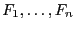in the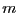unknowns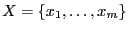such that the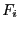may be written as: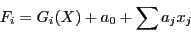is the sum of the non-linear function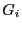and of the linear terms with coefficients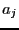. Let the interval evaluation ofbe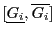: we define a new variable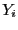as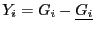, which imply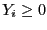.may now be written as the sum of linear term: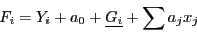Hence the system is now a linear system with the additional constraint that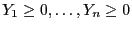. We may now apply a well-known method in linear programming: the simplex method which can be used to find the minimum or maximum of a linear function under the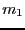equality constraints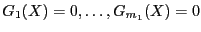and the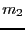inequality constraints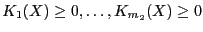. There are two phases in the simplex method: phase I verifies if a feasible solution exists and phase II is used to determine the extremum of the linear function.

In our case we may use only phase I or phase I and II by considering the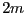optimum problems which are to determine the minimum and maximum of theunknowns under the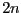constraints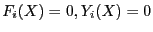and update the interval for an unknown if the simplex applied to minimize or maximize enable to improve the range. It may be seen that this is a recursive procedure: an improvement on one variable change the constraint equations and may thus change the result of the simplex method applied for determining the extremum of a variable which has already been considered.

This procedure, proposed in , enable to correct one of the drawback of the general solving procedures: each equation is considered independently and for given intervals for the unknowns two equations may have an interval evaluation that contain 0 although these equations cannot be canceled at the same time. The previous method enable to take into account at least partly the dependence of the equations. Clearly it will more efficient if the functions has a large number of linear terms and a "small" non-linear part.

In all of the following procedures the various storage mode and bisection mode of the general solving procedures may be used and inequalities are handled.Next: Implementation without gradient Up: Solving systems with linear Previous: Solving systems with linear   Contents
Jean-Pierre Merlet 2012-12-20